MEDIUM
DATA STRUCTURES AND ALGORITHMS

# Longest Common Subsequence (With Solutions in Python, Java & JavaScript)

Written ByTom WagnerChristopher Ang

## What is the Longest Common Subsequence Problem?

The Longest Common Subsequence (LCS) problem is a common technical interview question where you're asked to find the longest sequence of characters present in two strings. Variations of this problem are commonly found in real-world applications such as bioinformatics, natural language processing, and text comparison. This problem can be solved using dynamic programming techniques, which involve breaking down the problem into smaller subproblems and then solving them iteratively.

## Examples of the Longest Common Subsequence Problem

Given two strings, return the longest common subsequence between the two strings. A subsequence of a string is a string that contains characters from the original string in the same order as the original, but may have characters deleted.

#### Example 1

Input: s1 = "abccba", s2 = "abddba" Output: "abba"

#### Example 2

Input: s1 = "zfadeg", s2 = "cdfsdg" Output: "fdg"

#### Example 3

Constraints

• 1 <= s1.length, s2.length <= 1000

• There may be multiple valid answers, but they'll all have the same length.

## How to Solve the Longest Common Subsequence Problem

To solve the longest common subsequence problem (also known as longest common substring), it is helpful to first consider a couple of important properties of the `lcs` function. Firstly, if two strings `s1, s2` end in the same substring then their `lcs` is the `lcs` of the two strings without their common substring concatenated with said substring. For example, `lcs("abccba", "abddba") = lcs("abcc", "abdd") + "ba"`, since the length of the longest common subsequence between the two input strings is at minimum the length of the common consecutive string they share.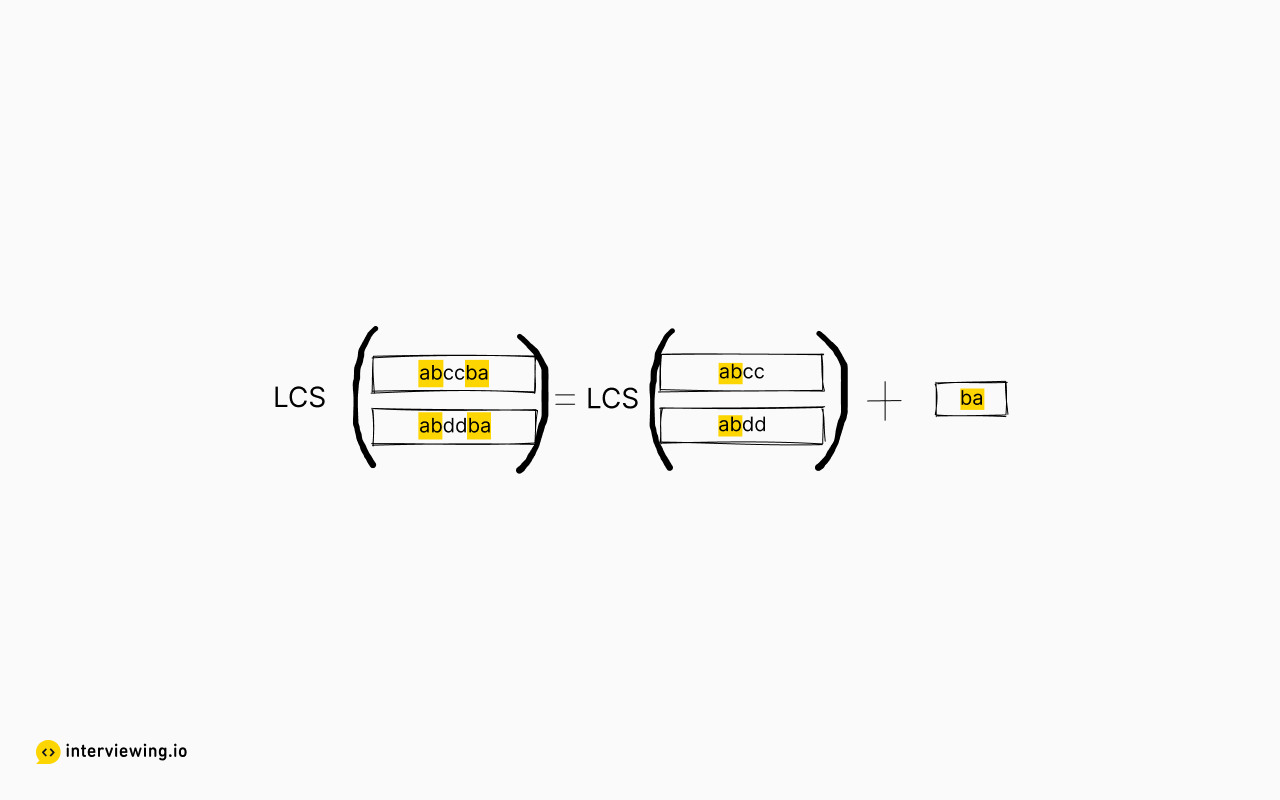Secondly, if two strings do not have a common ending substring, the `lcs` of the two strings will be the same as the `lcs` resulting from removing the ending of one of the strings. Put another way, `lcs(s1, s2)` is one of two recursive possibilities:

1. `lcs(s1[:-1], s2)`
2. `lcs(s1, s2[:-1])`

where `s1[:-1]` is a string with the last character removed. For example, suppose `s1="abcd", s2="zdef"`, then `lcs(s1, s2) = lcs("abcd", "zde") = lcs("abcd", "zd") = "d"`. But note in this example that `lcs("abcd", "zde")` is clearly not equal to `lcs("abc", "zde")`.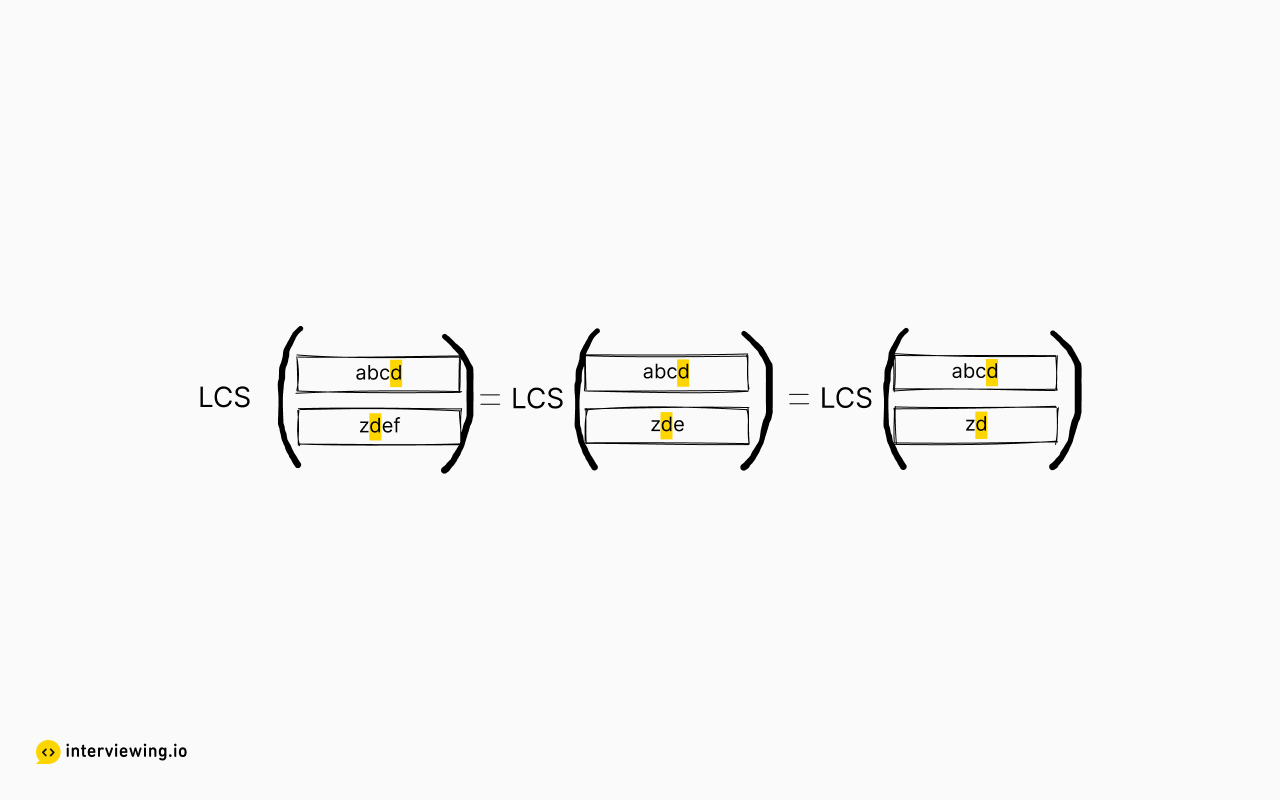### Recursive Approach

Leveraging the above two properties, we can use a recursive solution to approach a longest common subsequence algorithm and solve this using backtracking.

Starting at the end of the two strings:

1. If the characters at the end are the same, we can return `lcs(s1[:-1], s2[:-1]) + s1[-1]`.
2. If the characters are not the same, we must compute both `lcs(s1[:-1], s2)` and `lcs(s1, s2[:-1])`, and return the longer given sequence.
``````def solution(s1, s2):
if len(s1) is 0 or len(s2) is 0:
return ''
elif s1[-1] == s2[-1]:
return solution(s1[:-1], s2[:-1]) + s1[-1]
else:
sub1 = solution(s1[:-1], s2)
sub2 = solution(s1, s2[:-1])
return sub1 if len(sub1) > len(sub2) else sub2``````

#### Time/Space Complexity

Let `m` and `n` be the length of the two strings.

• Time Complexity: `O(2^m * 2^n)` in the worst case. This algorithm computes all possible subsequences for both strings, resulting in time complexity of `2^(len(s))` for a string, but it also computes all possible subsequences per subsequence of the other string, hence the product.
• Space Complexity: `O(max(m,n))`. The space complexity is due to the height of the recursion call stack being the maximum length between the two strings.

#### Recursive Solution With Memoization

When implementing a recursive algorithm, one optimization to always look out for is to address repeated work. For example, if we employ the above algorithm on a string that includes the substring "abc" in three different locations we will effectively be calling `lcs(abc)` three different times within the recursion tree. How can we avoid this and improve the time complexity? By storing the `lcs` computations in a lookup table, otherwise known as memoization.

``````def solution(s1, s2):
return solution_recur(s1, s2, {})
def solution_recur(s1, s2, solutions):
# use a frozenset here because (1) frozenset is hashable, so can be
# used for a key, and (2) order of the inputs does not matter for this function.
inputs = frozenset([s1, s2])
solved = solutions.get(inputs, None)
if solved is not None:
return solved
if len(s1) == 0 or len(s2) == 0:
solved = ''
elif s1[-1] == s2[-1]:
solved = solution_recur(s1[:-1], s2[:-1], solutions) + s1[-1]
else:
sub1 = solution_recur(s1[:-1], s2, solutions)
sub2 = solution_recur(s1, s2[:-1], solutions)
solved = sub1 if len(sub1) > len(sub2) else sub2
solutions[inputs] = solved
return solved``````

#### Time/Space Complexity

Due to how the recursive function is formulated, the `lcs` of `(m + 1) * (n + 1)` different input pairs will be computed. Since this solution caches those results, it has:

• Time Complexity: `O(m * n)`
• Space Complexity: `O(m * n)`

### Dynamic Programming Approach

Notice that in the previous approaches, we relied on the fact that the problem being solved had very similar subproblems which were used to ultimately solve the original problem. Of course, these types of situations can be tackled using memoization as seen above. However, an even more powerful approach can be used to tackle overlapping subproblems: dynamic programming.

To solve this using a dynamic programming approach, this solution will construct a table of results, and then trace back through the table from the bottom up to construct the longest subsequence. Letting `s1="abd"` and `s2="badc"`, the initial table would look like: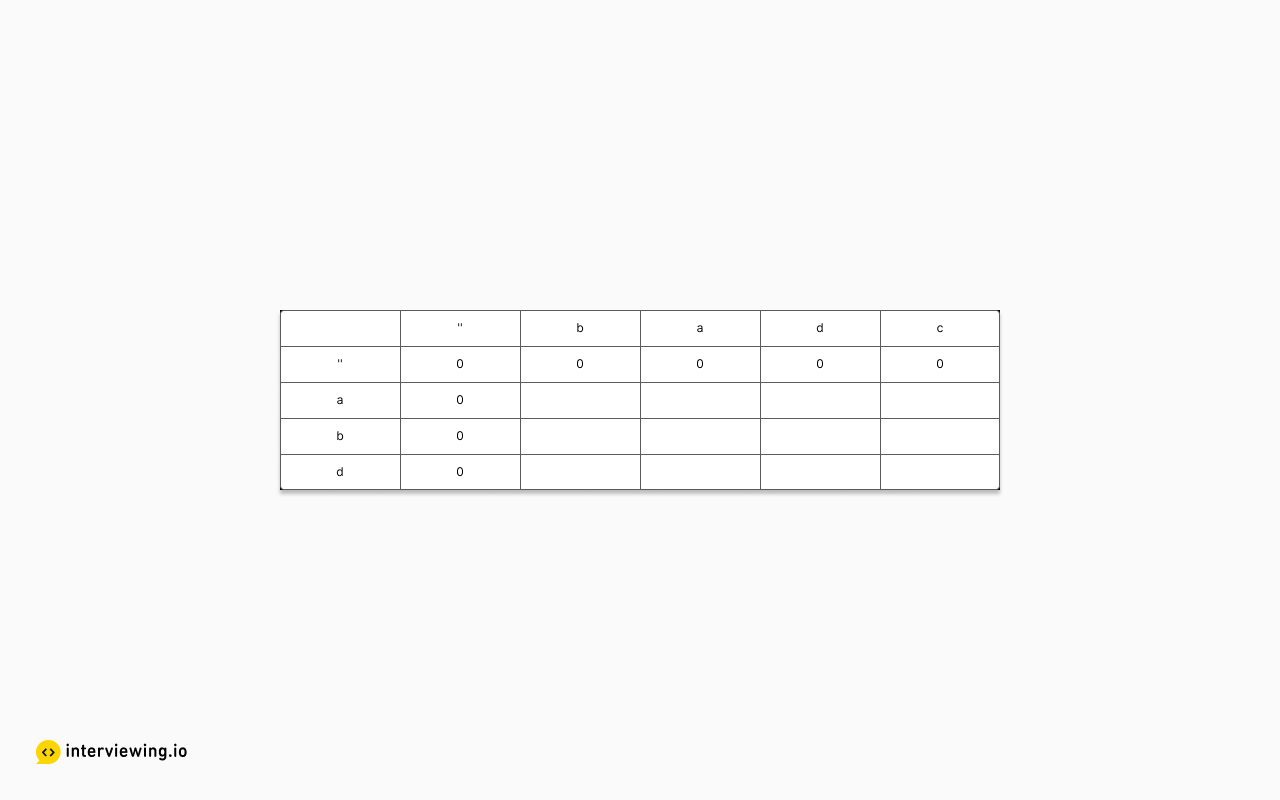Notice that the table has been augmented with a row and column for no characters in a string. The added row and column serve as a base case upon which to fill out the rest of the table.

The table can be constructed row by row (or column by column). At each cell the character of the row is compared with the character of the column, and based on the result the following items are stored:

1. The length of the longest subsequence between the two substrings up to and including the characters at the current cell. The value will be one plus the "previous" cell's value if the characters match; otherwise it will be the "previous" cell's value.
2. The direction of the cell "prior" to the current cell which has the largest value. If the characters match, then the "previous" cell is up one and to the left one. Otherwise it is whichever cell has the larger value between the cell to the left and the cell above.

Continuing with the example, to fill in the first row the following would occur:

1. 'a', 'b': unequal; above and to the left are 0; so store 0 with direction of "both"
2. 'a', 'a': equal; upper left is 0; so store 1 with direction of "up-left"
3. 'a', 'd': unequal; above is 0, but left is 1, so store 1 with direction of "left"
4. 'a', 'c': unequal; above is 0, but left is 1, so store 1 with direction of "left"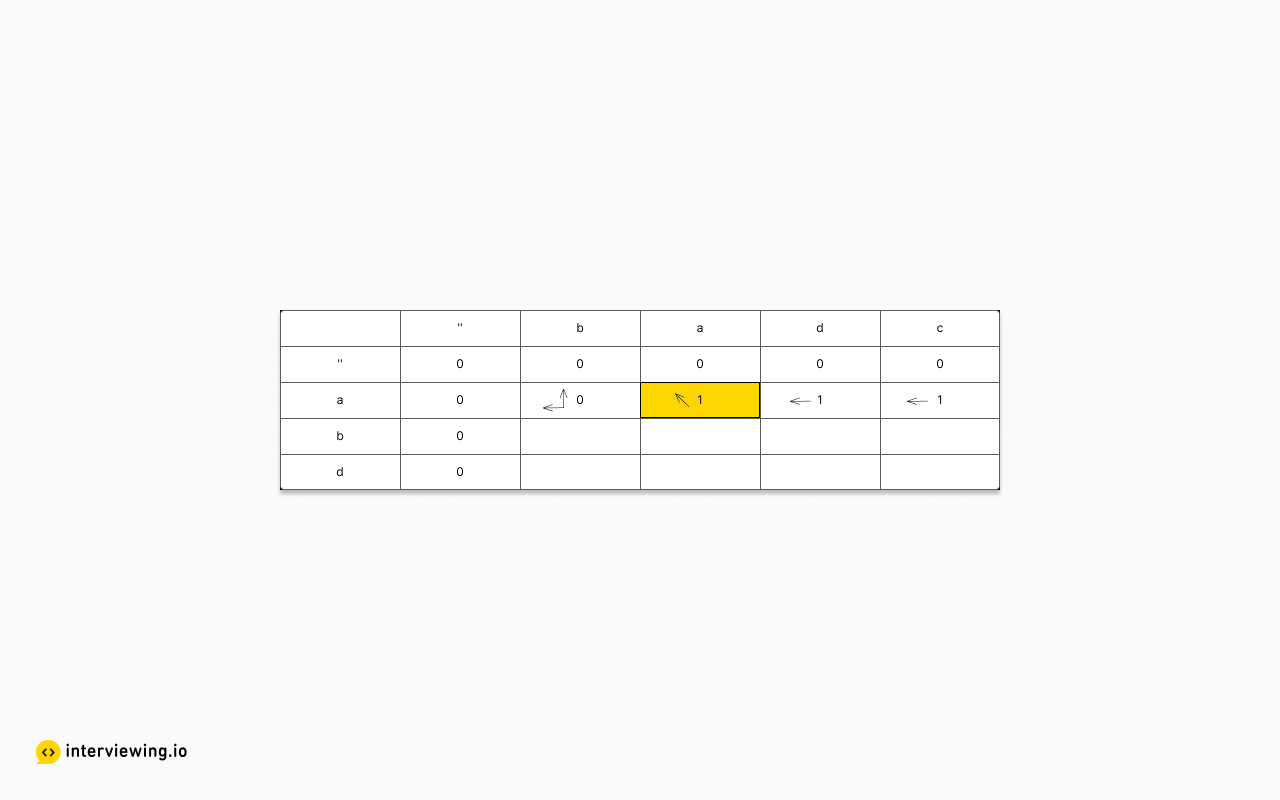Next we fill in the second row via:

1. 'b', 'b': equal; upper left is 0, so store 1 with direction of "up-left"
2. 'b', 'a': unequal; above and to the left are both 1; so store 1 with direction of "both"
3. 'b', 'd': unequal; above and to the left are both 1; so store 1 with direction of "both"
4. 'b', 'c': unequal; above and to the left are both 1; so store 1 with direction of "both"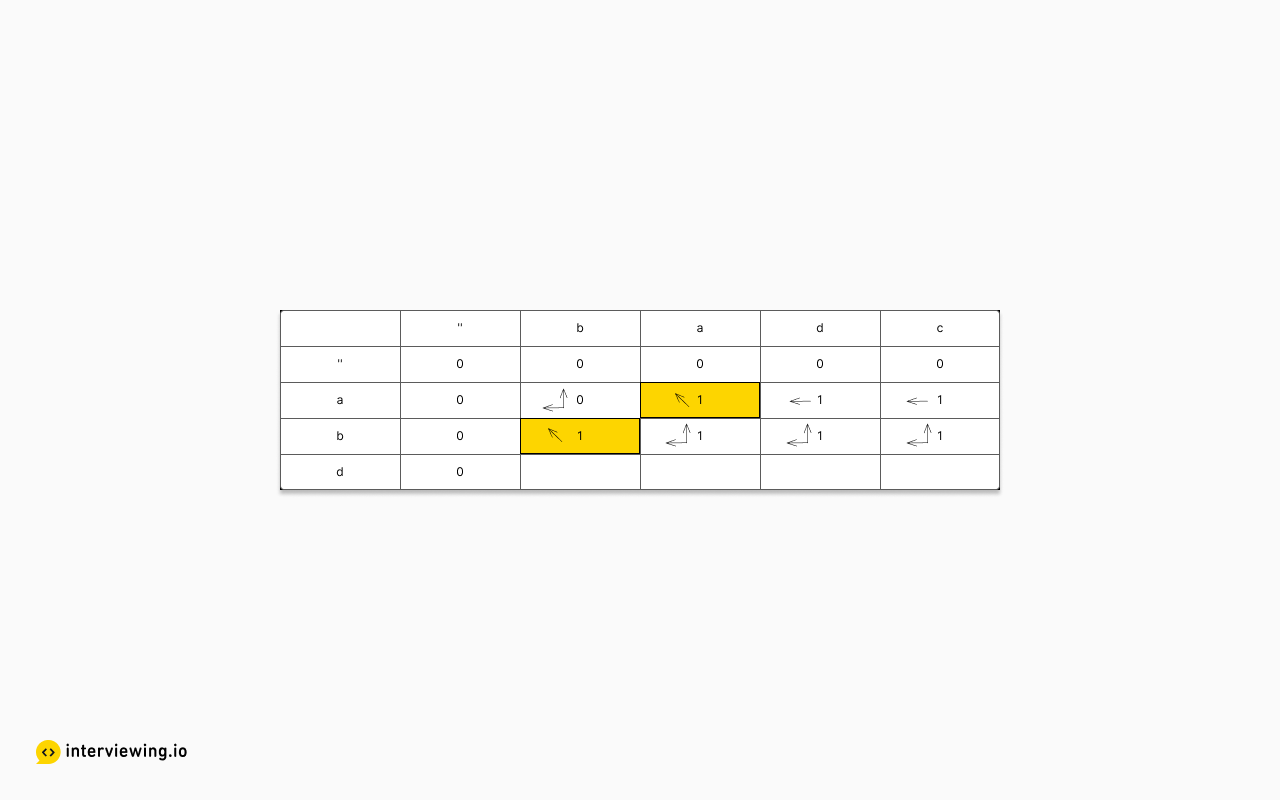Finally, the last row:

1. 'd', 'b': unequal; above and to the left are 0, so store 0 with direction of "both"
2. 'd', 'a': unequal; above is 1 while to the left is 0; so store 1 with direction of "up"
3. 'd', 'd': equal; upper left is 1; so store 2 with direction "up-left"
4. 'd', 'c': unequal; above is 1, while to the left is 2; so store 2 with direction 'left'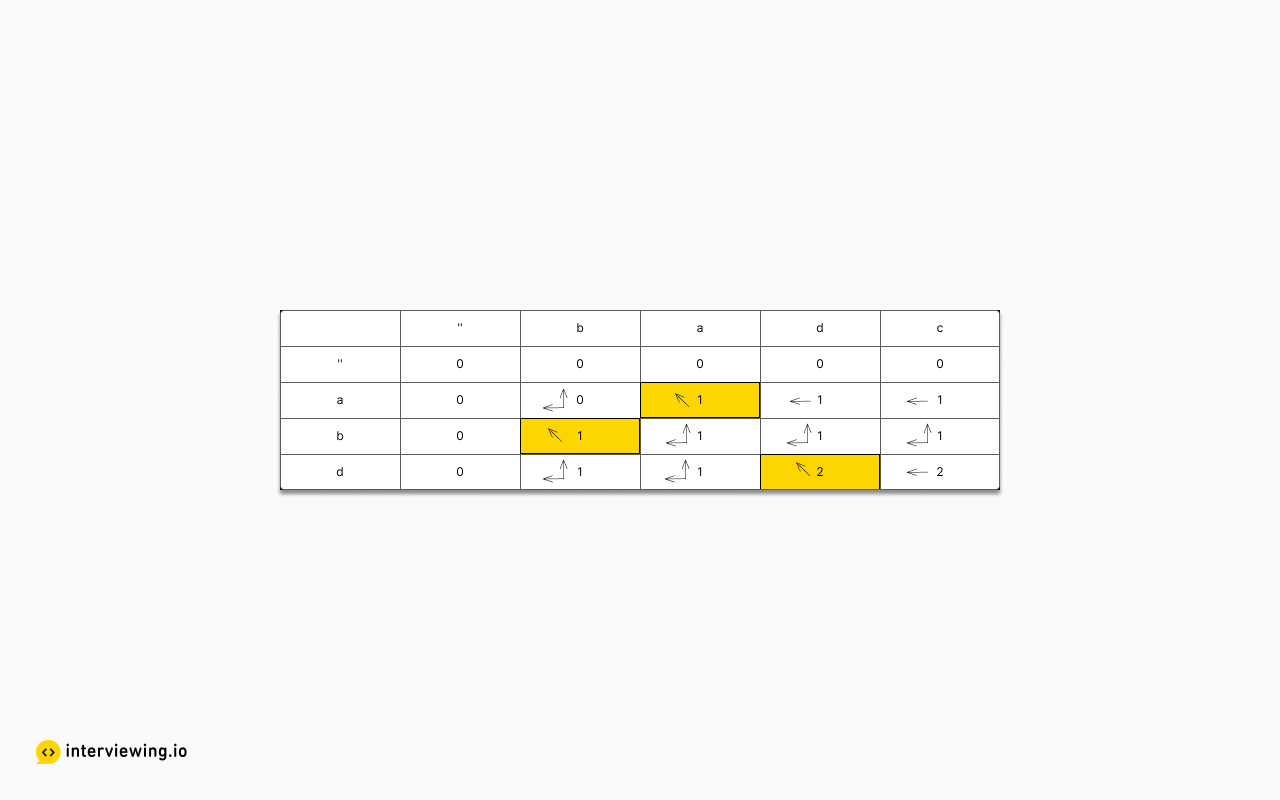Now that the table is completed we can trace back through the table to construct the `lcs`. To do so, we start in the bottom right corner of the table, which will have the largest number. At each cell, check the direction. If the direction is "up-left", then the character at the current cell is part of the `lcs`, so prepend it to the result (since the traceback goes through the table in reverse). Whether the character is part of the `lcs` or not, follow the direction encoded in the cell to navigate the table until the value of the cell is zero.

Using the example above, the traceback would proceed as follows:

1. Start in the bottom right corner, where the value stored is 2. This indicates any `lcs` has a length of 2. As the direction is `left`, move to the cell `(d,d)`
2. In the cell `(d,d)`, the direction is `up-left`, so `'d'` is the last character in an `lcs`. Prepend `'d'` to the result, which is then just `"d"` so far, and move to the cell `(b,a)`.
3. In the cell `(b,a)`, the direction is `both`, and the algorithm could choose to go either to the `left` or `up` (with different resulting strings). For this example, the canonical direction is `up`, so navigate to the cell `(a,a)`.
4. In cell `(a,a)`, the direction is `up-left`, so `'a'` is the penultimate character in an `lcs`. Prepend `'a'` to the result to end up with a string of `"ad"` so far, and move to the cell `('',b)`.
5. In the cell `('',b)`, the value stored is 0, so the algorithm stops, and the `lcs` is `"ad"`.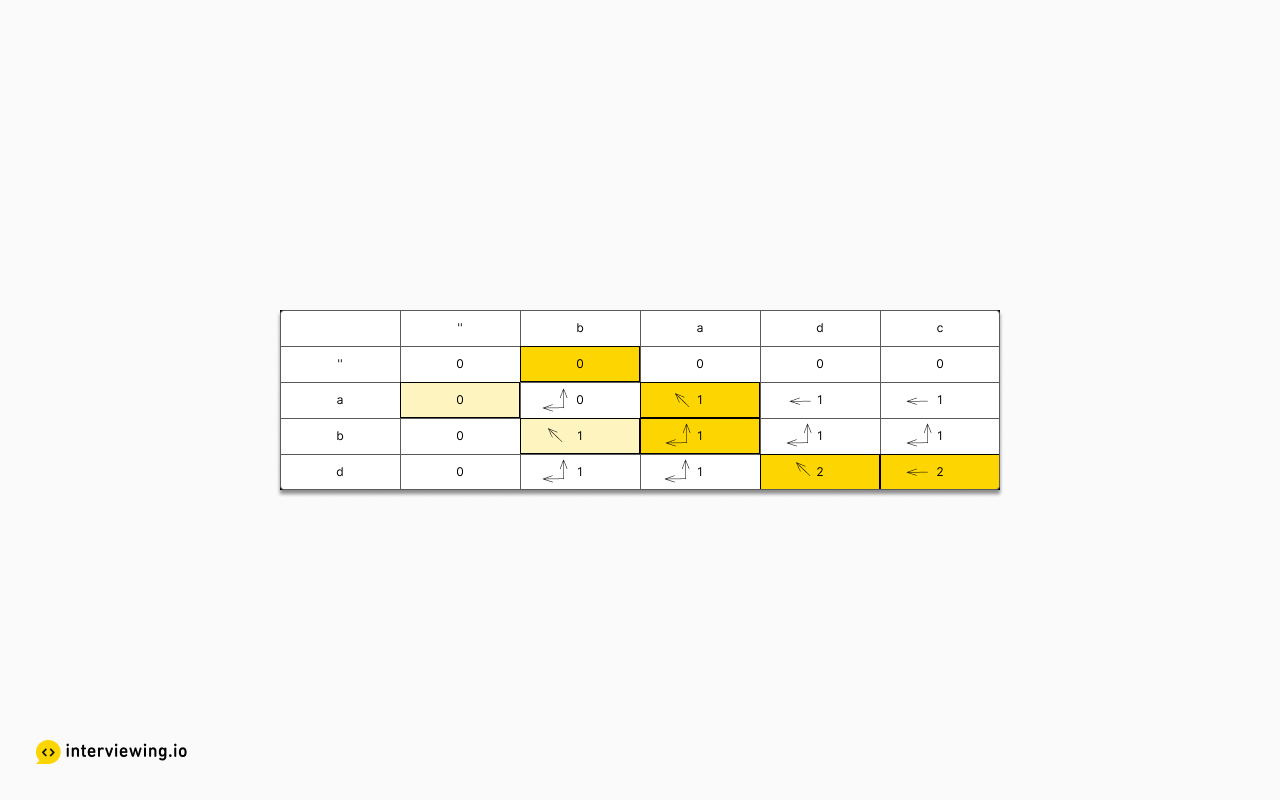Note 1: In step 3, had the algorithm gone to the `left` instead of `up`, the `lcs` would have been `"bd"` instead of `"ad"`.

Note 2: It is possible to store the actual subsequences inside each cell, but such a solution would need to include all subsequences that could be arrived at in each cell, which would add to the space complexity.

``````class SolutionNode:
def __init__(self, direction="sink", value=0):
# valid directions are: sink, up, left, up-left, or both
self.direction = direction
self.value = value
def solution(s1, s2):
if len(s1) == 0 or len(s2) == 0:
return ''
# building the table with the extra row and column
lcs = [[SolutionNode() for x in range(len(s2)+1)]
for y in range(len(s1)+1)]
# skip first row; it is supposed to be all zeros anyway
for i, row in enumerate(lcs[1:], 1):
# skip first row; it is supposed to be all zeros anyway
for j, cell in enumerate(row[1:], 1):
if s1[i-1] == s2[j-1]:
cell.value = lcs[i-1][j-1].value + 1
cell.direction = 'up-left'
elif lcs[i][j-1].value == lcs[i-1][j].value:
cell.direction = 'both'
cell.value = lcs[i][j-1].value
elif lcs[i][j-1].value > lcs[i-1][j].value:
cell.direction = 'left'
cell.value = lcs[i][j-1].value
else:
cell.direction = 'up'
cell.value = lcs[i-1][j].value
# The table is built; now to traceback
i = len(s1)
j = len(s2)
node = lcs[i][j]
val = node.value
result = ''
while val > 0:
# Could instead go 'left' on 'both'
if node.direction == 'up' or node.direction == 'both':
i -= 1
elif node.direction == 'left':
j -= 1
else:
i -= 1
j -= 1
# need to prepend since this constructs the lcs in reverse
result = s1[i] + result
node = lcs[i][j]
val = node.value
return result``````

#### Time/Space Complexity

• Time Complexity: `O(m * n)`.
• Space Complexity: `O(m * n)`.

The final solution can be further improved. One such way is the Hunt-Szymanski Algorithm.

## Practice the Longest Common Subsequence Problem With Our AI Interviewer

Start AI Interview

## Longest Common Subsequence Frequently Asked Questions (FAQ)

### Which approach to solving longest common subsequence is the most efficient?

The optimal time complexity of the longest common subsequence (LCS) algorithm is `O(m * n)`, where `m`and `n` are the lengths of the input strings. This is typically implemented using dynamic programming, where we create a matrix of size (m+1) x (n+1) to store the prefix lengths and fill the matrix iteratively, requiring examining each cell, which takes constant time. Another way to achieve this time complexity is using recursion with memoization, where we ensure no duplicate computations are made when visiting each node in the resursive tree. As a result, the overall time complexity is determined by the number of cells in the matrix or nodes in the recursive tree.

### What is the brute force approach to solving the longest common sequence problem?

The longest common subsequence (LCS) algorithm can be solved naively with recursion - the algorithm exhaustively explores all possible combinations of characters in both strings and returns the longest length. Without cacheing, this results in a large number of redundant computations, which leads to an exponential time complexity - `O(2^(m + n))`, where m and n are the lengths of the input strings. This inefficiency makes the brute force recursive approach impractical for larger input sizes.

### Can there be more than one longest common subsequence?

No, by definition, there can only be one longest common subsequence (LCS) between two strings. The LCS is defined as the longest subsequence that is common to both strings, meaning it appears in both strings in the same order but not necessarily consecutively.